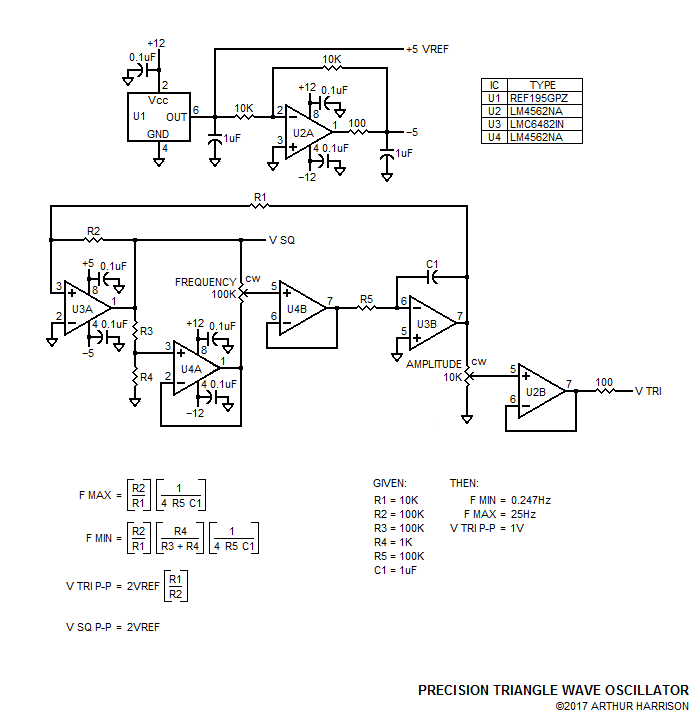Precision Triangle Wave Oscillator

The circuit illustrated provides precise low-frequency triangle waves with excellent linearity and symmetry with parameters that closely follow the equations provided.

U1 is a +5V reference that requires capacitive loading (1uF) for stability. This capacitance also provides the advantage of excellent transient response, as well as lowered noise. U2A is a low-noise operational amplifier configured for a gain of -1, with a 1uF capacitor providing further noise reduction. The 100 ohm resistor at U2A's output prevents the capacitor from causing op-amp instability.

The +5 and -5 volt outputs from U1 and U2A provide the supplies for dual op-amp U3. U3A is configured as a comparator with a reference of 0V at its negative input. U3B is configured as an integrator. U3 utilizes CMOS devices, permitting the comparator's lightly-loaded output to swing essentially to the +5 and -5 volt supply rails, contributing to waveform symmetry.

Resistors R3 and R4 divide the V SQ voltage by about 100, driving the input of unity-gain configured U4A. A resulting square wave with amplitudes of about +0.1 and -0.1 volt result at U4B's output.

The square waves are applied to the clockwise and counterclockwise terminals of a 100K potentiometer, the slider of which drives the input of unity-gain configured op amp U4B. U4B's output drives the U3B integrator, which exhibits a time constant determined by R5 and C1. R5 may be a relatively large value, since the input current of CMOS U3 is very small, preventing any significant offset voltage.

The output of U3B is fed back to the comparator section, U3A, through resistor R1, completing the oscillator's positive feedback loop. Operational amplifier sections U4A and B, while not strictly necessary for oscillation, provide the advantage of removing the frequency potentiometer's end-to-end tolerance from the frequency-determining equation. This permits the use of any potentiometer, provided that it has a relatively high value to allow U3A's output to swing close to the rails. An inexpensive ten-turn type such as Bourns 3590S-2-104L, equipped with a turns-counting dial, would therefore be suitable for an accurate direct-indication of frequency.

U3B's output drives an amplitude potentiometer buffered with unity-gain configured op-amp U2B, permitting the triangle output amplitude to be set between 0 and 1V P-P.

The values shown provide an approximate 100:1 frequency range of 0.247Hz to 25Hz. Note that R3 and R4's ratio may be refined for an attenuation of precisely 100:1 to obtain a more precise lower value of 0.25Hz (where R3 is made a non-standard value of 99K ohms).

While the circuit's frequency may be predicted by the equations at relatively low frequencies, propagation delays, comparator recovery time, and stray reactance will degrade that predictability as the integrator's time constant is lowered. The practical limit of F MAX is about 250Hz, where a measured -3% deviation from the predicted value occurs.

The oscillator's frequency range may be conveniently switched in decade bands by substituting C1 with larger values (to lower the frequency) or smaller values (to raise the frequency). A metalized polypropylene or similar low-loss capacitor should be used for C1.November 9, 2017

Source documents dated October 23, 2017

Text and image ©2017 by Arthur Harrison